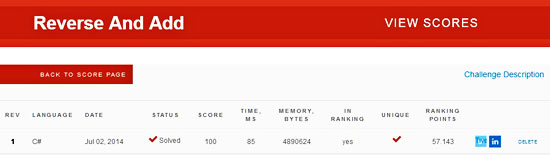# CodeEval 3 – Reverse And Add

April 30, 2015CodeEval is a series of posts which are different than the typical engineering/Excel/VBA posts that are being published in this blog. The purpose of this series is to demonstrate possible solutions to various CodeEval programming challenges. Each solution has already been submitted and accepted as valid on CodeEval platform, so if you try to submit the presented solution as it is, you will probably get a “not unique solution” result. The solutions will be presented in the C# language, but the logic/algorithm behind them is similar despite the language you might use.

### Reverse And Add – challenge description

Choose a number, reverse its digits and add it to the original. If the sum is not a palindrome (which means, it is not the same number from left to right and right to left), repeat this procedure.

Example:

195 (initial number) + 591 (reverse of initial number) = 786

786 + 687 = 1473

1473 + 3741 = 5214

5214 + 4125 = 9339 (palindrome)

In this particular case, the palindrome 9339 appeared after the 4th addition. This method leads to palindromes in a few steps for almost all of the integers. But there are interesting exceptions. 196 is the first number for which no palindrome has been found. It is not proven though, that there is no such a palindrome.

#### Input sample

Your program should accept as its first argument a path to a file name. Each line in this file is one test case. Each test case will contain an integer n < 10,000. Assume each test case will always have an answer and that it is computable with less than 100 iterations (additions).

#### Output sample

For each line of input, generate a line of output which is the number of iterations (additions) to compute the palindrome and the resulting palindrome. (they should be on one line and separated by a single space character). Example:

4 9339

The particular challenge has a relatively low success rate (66.6% – 30/04/2015), and its level of difficulty is medium. More info you can find here (update: the CodeEval has shut down, so the link was removed).

### Solution

Apart from the main code, the solution incorporates two helper methods for reversing the input number (“ReverseNumber”) and for checking if a number is a palindrome (“IsPalindrome”).

``````using System;
using System.Collections.Generic;
using System.IO;
using System.Linq;
using System.Text;

/*
------------------------------------------------------------------
The code below solves the CodeEval challenge -  Reverse And Add.

Written by:    Christos Samaras
Date:          02/07/2014
e-mail:        [email protected]
site:          http://www.myengineeringworld.net
------------------------------------------------------------------
*/

{
class Program
{
static void Main(string[] args)
{
{
{
if (line != null)
{
int cnt = 0;

while (IsPalindrome(line) == false)
{
int reversedNumber = int.Parse(ReverseNumber(line));
line = (int.Parse(line) + reversedNumber).ToString();
cnt++;
}
Console.WriteLine(cnt + " " + line);
}
}
}
}

public static string ReverseNumber(string number)
{
char[] charArray = number.ToCharArray();
Array.Reverse(charArray);
return new string(charArray);
}

public static bool IsPalindrome(string num)
{
if (num == ReverseNumber(num))
return true;
else
return false;
}
}
}

/* NOTE: the solution has already been submitted and accepted as valid! */``````

### Points

Here is proof that the solution works and the points given by the CodeEval platform.Page last updated: 17/06/2019

Category: CodeEval